# News

## MS Math – Week of Oct 25-286th Grade Math – Mrs. Evans

This week we will finish Chapter 2 with a test on Tuesday.   This test focuses, largely, on multiplying and dividing fractions and mixed numbers.  For students who need additional help or practice with these skills, please contact Mrs. Evan s or go to IXL.COM – sections H and I.

Beginning on Wednesday we will be learning about how we can represent real world quantities as negative integers and rational numbers.  It is useful to be able to represent every situation as either positive or negative in order to think of them in a mathematical way.  We will spend time making sure that we can order positive and negative rational numbers properly before we put them into our tool box for all our future use!

Math Grade Math – Mrs. Evans and Mrs. VonFeldt

This week we will finish Module 3, which is also the conclusion of Unit 1.  Upon completion of this unit, students will have the ability to use rational numbers (fractions, decimals and integers) that are both negative and positive, in all the basic operations.  They will have practiced, in lesson 3.6, this week, to apply a strategy that will help them in solving real world problems that deal with both decimals and fractions. The Unit 1 Test will be this Friday. For any students who feel the need for additional practice on the skills we have learned in Unit 1, please go to IXL.COM – section H.

8th Grade Math – Mrs. Ernest

Students have been learning how to graph from an equation or a data table. This week we will be expanding our knowledge of linear equations, functions in general and the coordinate plane.

https://www.cuemath.com/algebra/linear-equations/

Algebra – Mrs. VonFeldt

We are continuing to explore the question: How can we use linear relationships to solve problems? A graph is one representation of a function. This week, students will learn to graph linear equations using two different strategies: 1) make a table and 2) graph using intercepts. If the x and y-intercepts are known, or can be easily found, then a straight line going through those points makes a line: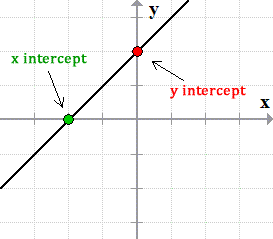We will also begin exploring slope. What is slope and how can you calculate it between two points? This will lead us into the slope-intercept form next week.## MS Math – October 18-226th Grade Math – Mrs. Evans

This week we will be dividing fractions and mixed numbers.  Dividing fractions is actually a pretty simple thing to do, especially after we know how to multiply fractions.  This is because a division can be considered the same as multiplying by the reciprocal.  A reciprocal is just the “flip” of a number or fraction.  For example the reciprocal of 3/5 is 5/3.  The numerators and denominators just trade places.  We have a great way to remember how to divide by fractions:When dividing with a mixed number, just as we do in multiplying, we switch the mixed number into an improper fraction and then continue as if it were any other fraction.  Here is a reminder for those who need help converting a mixed number into an improper fraction: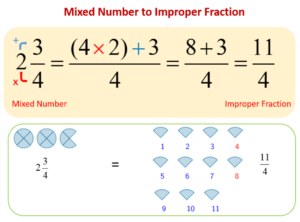We will practice being able to apply our new skills in problem solving as we collaborate with each other.  We will also focus on being able to verbalize how to multiply and divide fractions.  We will finish the week with a KAHOOT covering all the topics from Chapter 2.

Math Grade Math – Mrs. Evans and Mrs. VonFeldt

This week we will continue working in Module 3, not only adding and subtracting positive and negative rational numbers but multiplying and dividing them.  These are foundational skills requiring a degree of fluency.  Students who are struggling with these skills should seek help so that they are confident as the move into algebra later in the year.  A great place for extra help with immediate feedback on how a student is doing, is http://IXL.COM

The rules we learned with integers that help us to multiply and divide are still applicable with rational numbers.  Here are the rules to remind us:8th Grade Math – Mrs. Ernest

This week students will be refining their graphing skills, practice finding the difference between points, and interpret graphs through word problems. Students struggling with graphing skills should revisit these IXL activities:Algebra – Mrs. VonFeldt

This week, we will finish up Chapter 2 with a test on Tuesday. At the end of the week, students will study a roller coaster problem. They will take data, make a table of values and a graph to evaluate their results and determine if the coaster is safe for tall riders. This will introduce linear functions and graphs, which will be the focus of Chapter 3.

## MS Math – Oct 11-15, 20216th Grade Math – Mrs. Evans

This week we will be extending our understanding and skills with fraction operations, beginning with writing decimals as fractions and fractions with decimals.  We will compare and order decimals and fractions and then we will begin to multiply fractions, learning a wonderful trick to simplify them as we multiply.  Here is a little picture to help students, and families, convert between decimals and fractions:Converting from a decimal to a fraction is done by remembering that a decimal is a portion of a factor of ten, as shown here:We understand that we can write a decimal as a fraction and a fraction as a decimal simply by remembering these simple lessons.  Here is a video that can help students who want a little more help converting from a decimal to a fraction and a fraction to a decimal:

Math Grade Math – Mrs. Evans and Mrs. VonFeldt

This week we will be working in Module 3, in which we will apply all that we learned in Modules 1 and 2 to rational numbers.  Rational numbers are any number that can be represented by a ratio of two integers (the second of which is not zero).  The most important thing to know about rational numbers is that they include decimals and fractions.  Here is a picture showing what rational numbers are:We apply the rules and logic we learned about operations with negatives to decimals and fractions.  This means we will be able to do all the basic operations to all the types of numbers!    For any students who want to review how to convert between decimals and fractions, please see the video links above (6th grade math update).

8th Grade Math – Mrs. Ernest

This week 8th graders are reviewing algebra concepts from last week and applying these ideas to graphing problems. We will be working through Chapter 2 this week, focusing on how to graph, how to graph linear equations from an x,y table and writing linear equations from word problems.

Graphing Review:

Algebra – Mrs. VonFeldt

This week, students will continue to solve and master solving one-variable equations. They will learn how to solve proportional relationships using cross products: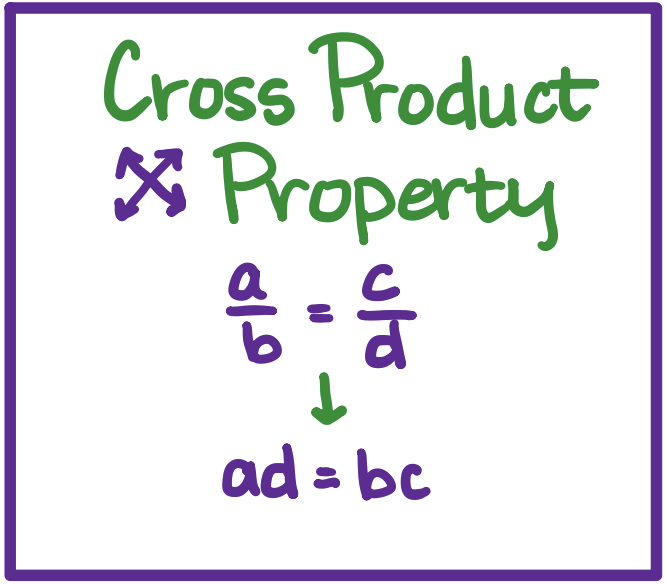We will also learn how to rewrite equations and formulas to be solved for a specific variable. This is often helpful to rewrite formulas with multiple variable inputs. At the end of the week, we will wrap up Chapter 2 with a team test on Thursday. The individual test will be next Tuesday.

## MS Math – Oct 4-8, 2021

1.6th Grade Math – Mrs. Evans

This week we will finish Chapter 1 with a test on Wednesday.   We will carry with us the ability to do all decimal arithmetic!  For help with decimal arithmetic, see the link to the Math Antics video in last week’s post.   Also in this chapter we learned about finding the least common multiple (LCM) and greatest common factor (GCF) and how to use these values to solve real world problems.  One of the important properties that we have reviewed in this chapter is the distributive property.  This is a property that we will use a great deal in algebra.  The distributive property allows us to distribute a factor across a sum.  Here is how it looks: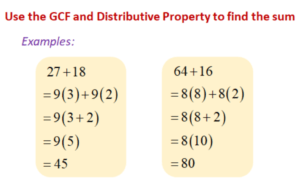After we finish with the Chapter 1 test, we will continue to learn more about decimals in Chapter 2.

Math Grade Math – Mrs. Evans and Mrs. VonFeldt

This week we will review and test on Module 2 which covers multiplying and dividing integers.  If we remember the rules (shown in last week’s post), it can be a straight forward process.  It is, however, necessary that we continue to ALWAYS use the proper order of operations.  Here is a reminder of PEMDAS which helps us keep  the order correct: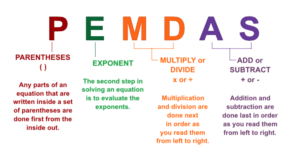For students who need help with Module 2 concepts, here is a video summarizing how we multiply and divide integers.

When we finish with the Module 2 test, we will begin to apply what we have learned about negatives to rational numbers (fractions, decimals and mixed numbers).

8th Grade Math – Mrs. Ernest

This week students will begin solving multi-step algebraic equations. Students will be working through the remainder of Chapter 1, and we will begin Chapter 2 by the end of this week. I will be specifically looking for each student to write out their work as clearly as possible! See the image below for an example one-step problem and the requirements for showing work on homework.Algebra – Mrs. VonFeldt

This week is all about solving linear equations. By the end of the week, students should be able to solve all types of one variable equations using the following steps:

• Distribute;
• Combine like terms;
• Inverse multiplication/division.

Along the way, students will learn to organize and show their work on each step. The following video walks through this process with a number of different types of equations:

## MS Math – Sept 27 – Oct 16th Grade Math – Mrs. Evans

This week we will be investigating DECIMALS! We will begin with a review of how we add and subtract decimals (line up the place values) and then we will learn to multiply and divide them.  Here is a video that reviews all that we will work on this week.

Math Grade Math – Mrs. Evans and Mrs. VonFeldt

This week we will continue to learn about using integers as we learn to multiply and divide positive and negative integers.  We will practice modeling and establish some great rules that apply to multiplying and dividing integers.  Here is a summary of the rules we use when multiplying and dividing integers:8th Grade Math – Mrs. Ernest

This week 8th graders will be learning to solve one and two step algebraic expressions using our rules for adding, subtracting, dividing, and multiplying rational numbers (decimals and fractions). Students will need to be proficient with the skills we have been learning so far this year. If your student is feeling confused by the concepts we’ve covered so far, make sure they practice with these IXL activities! We will cover Chapter 1-5, 1-6 and part of 1-7 to wrap up this unit this week. Next week we will have a unit test on all topics covered this year so far!

Algebra – Mrs. VonFeldt

This week we are learning about functions. A function is a relationship that consists of a set of inputs, called the domain, and a set of outputs, called the range. Each input can be paired with only one output. We will spend time looking at different representations of functions such as verbal models, tables, graphs and equations. This will bring us to the end of Chapter 1. There will be a test on Friday. The following video is a good review of functions and how to name them:

## MS Math Post – Sept 20-24, 2021Be careful!  Don’t divide by zero!   🙂

This week we will miss a few math classes due to MAP testing.

6th Grade Math – Mrs. Evans

This week we will apply what we learned last week – finding the greatest common factor (GCF) and least common multiple (LCM).  We will use the GCF to rewrite products with the distribution property.  This is a tool that we will use often in algebra!    For students needing help with LCM or GCF, please visit last weeks video links.  Here is how finding the GCF can help us in using the distributive property.

Math Grade Math – Mrs. Evans and Mrs. VonFeldt

This week we will practice adding and subtracting integers.  This skill is very important so we will make sure we have mastered it before moving on!  See last week’s post for a Math Antics video for some help.    One of the important lessons that we will apply this week is that, because addition and subtraction are opposites, we can rewrite problems like this:

8 – 9 = 8 + (-9)

8 – (-9)  =  8 + 9

There are various strategies that we can use to help us add integers of same or different signs.  We can use counters, number lines or absolute values.

8th Grade Math – Mrs. Ernest

This week the 8th graders are missing some of our classes due to Map testing, but that won’t stop them from demonstrating all they’ve learned about rational numbers in a short quiz! We have been reviewing multiplying, dividing, adding, subtracting and converting fractions. We have also grown more comfortable switching between fractions and decimals. Towards the end of this week, we will wrap up Chapter 1.1-1.4 and introduce concepts from Chapter 1.5.

Here are some review resources:
Word problems with fractions:

Algebra – Mrs. VonFeldt

This week we explore what it means for one measurement to be more or less precise than another. This leads to a discussion of significant digits. Significant digits are the digits in a measurement that carry meaning contributing to the precision of the measurement. Students will learn the rules for adding/subtracting and multiplying/dividing using significant figures. This is especially important in today’s world of calculators that give values that go on and on and on…. Where should we stop and round our answers? Watch the following video for examples of why significant figures are useful:

We will also begin to look at mathematical relationships as functions. A function is a relationship in which each input is paired with only one output. The set of inputs of a function is called the domain of the function. The set of outputs is call the range. We will continue with our study of functions next week.

## MS Math Week of Sept 13-17We are looking forward to ZOOM Curriculum Night on Wednesday at 6:30 pm!

6th Grade Math – Mrs. Evans

This week we will be learning about prime factorization, finding the least common multiple and the greatest common factor.  These are foundational skills which will serve us well as we build new concepts to help us solve problems.  Our math books should be here soon, but for those who need a little extra help here is a support video on each of those important concepts:

PRIME FACTORIZATION

LEAST COMMON MULTIPLE

GREATEST COMMON FACTOR

7th Grade Math – Mrs. Evans and Mrs. VonFeldt

This week we will continue to develop our ability to work with negative numbers and by the end of the week we will be able to add and subtract any integer.  The use of number lines and counters (in our mental space) can really help solve problems like these.  It is good to be reminded that SUBTRACTION IS THE OPPOSITE OF ADDITION.  This means that subtraction is just adding the opposite (negative) and subtracting a negative number is the same as adding that number.  For example:

6 – 9 = 6 + (-9)

9 + (-2)  = 9 – 2

7 – (-3) = 7 + 3

For those who would like a little video support on this topic, here is a link to a great video:

8th Grade Math – Mrs. Ernest

This week we will review techniques from last week to identify least and greatest common multiples (see 6th grade math post above for some reminder videos on these topics). We will advance our understanding of multiplying and dividing fractions (Chapter 1 Lesson 2).

Ex: How do I multiply a mixed number by an integer?

To multiply a mixed number by an integer there are two efficient strategies. The first is by looking at the calculation as repeated addition.

For example: 2 1/3 x 3 = 2 1/3 + 2 1/3 + 2 1/3

You then add the whole separately and then the parts and then combine to get the final answer.

2 x 3 = 6.

1/3 x 3 = 3/3 = 1

6 + 1 = 7

The second involves converting the mixed number to an improper fraction.

2 1/3 = 7/3

7/3 x 3 = 21/3 = 7

Multiplying Fractions Review:

Algebra – Mrs. VonFeldt

This week, we will focus on translating verbal phrases and situations into equations and inequalities. This is a very important skill to develop in order to become a proficient problem solver. Key words can be used to identify the intended operation, such as “more than” for addition, “difference of” for subtraction, or “shared between” for division. It can also be helpful to draw a diagram to represent what you are trying to find. Variables can represent any quantities that are unknown.

We will also use dimensional analysis to convert units and rates. Here is a helpful video that shows how to use dimensional analysis to for unit conversion and problem solving:

## Middle School Math – Week of Sept 6th-10th, 2021Welcome to the Middle School Math Weekly Post.  It is our hope that this post will help parents and students to have a great math year!  You will find, in the posts, an update on what we are currently working on and often some other helps like videos and pictures.

6th Grade Math – Mrs. Evans

This week we will begin to work multi-digit division and prime factorization.  For students who want a review of long division, you can watch this video:

7th Grade Math – Mrs. Evans and Mrs. VonFeldt

This week we will begin to learn how to do operations fluently using negative numbers  We will begin in Module 1 of our GO MATH books.  We will be thinking about operations with negatives in a variety of ways so that it becomes comfortable and innate.  First, we add integers with a common sign and then we will learn to add integers with opposite signs. As you practice, look for patterns to make the computations easier.

8th Grade Math – Mrs. Ernest

This week we will begin to learn how to confidently convert between fractions and decimals. Students will gain confidence with percentages, ratios, and conversions. We will be working through Chapter 1, sections 1, 2, and 3 this week, given the time.

IXL in class practice:
Practice the Kahoot from class:
Review Mixed numbers to Improper Fractions:

Review improper fractions to mixed numbers:

Algebra – Mrs. VonFeldt

This week students will review and master some of the foundational skills that will be used as we evaluate algebraic expressions and equations. Remember to always use the correct order of operations and avoid those common mistakes!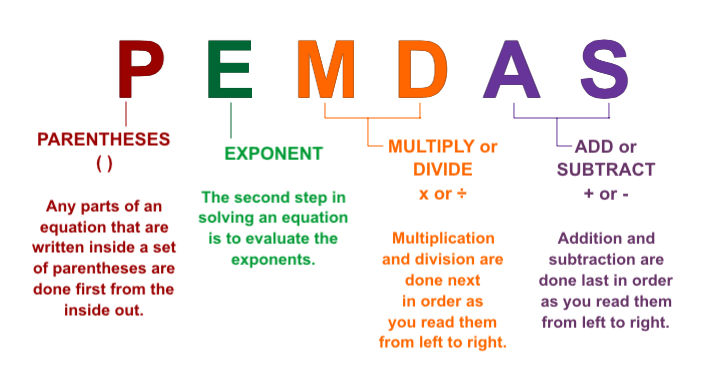We will finish the week with a fun CSI activity using real numbers to solve puzzles.

© 2022 Assumption Catholic School | 2116 Cornwall Ave, Bellingham, WA 98225
Phone: 360.733.6133 | Fax: 360.647.4372
Email: theoffice@school.assumption.org
Dashboard | Web design and development by Olywebdev.com